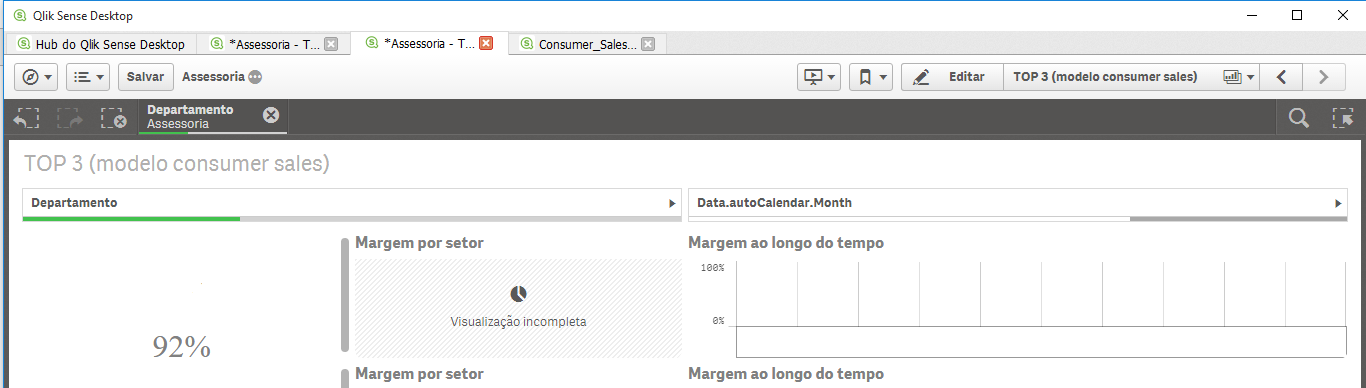Announcements
The way to achieve your own success is the willingness to help somebody else. Go for it!
cancel
Showing results for
Did you mean:Not applicable

## Client with third largest sales

Hi,

I'm trying to create a KPI for each one of the top3 clients by margin. I've so far managed to create a bar chart with the clients with the top 3 margins. But the (slightly) same code won't work for a KPI.

Here's the (working) code for the measure in the bar chart:

if(rank((sum(Valor) - sum([Custo]*Horas))/sum(Valor))<=3,(sum(Valor) - sum([Custo]*Horas))/sum(Valor))

And here's the one I've been trying to make work on a KPI:

if(rank((sum(Valor) - sum([Custo]*Horas))/sum(Valor))=3,(sum(Valor) - sum([Custo]*Horas))/sum(Valor))

The problem is the KPI is always "-" no matter what value or expression I put in the code above. I assume there's a misunderstanding in how the aggr function works on my part, but couldn't tell where the error is coming from. Can someone help me?

1 Solution

Accepted SolutionsMVP

Can you try this:

Max(Aggr((Sum(Valor) - Sum([Custo]*Horas))/Sum(Valor), Client), 3)

and may be this to get the name of the 3rd larget client

FirstSortedValue(Client, -Aggr((Sum(Valor) - Sum([Custo]*Horas))/Sum(Valor), Client), 3)

9 RepliesMVP

I can't spot the aggr() function in your code, have you posted the correct code snippet?MVP

Try maybe

Sum( Aggr(

if(rank((sum(Valor) - sum([Custo]*Horas))/sum(Valor))<=3,(sum(Valor) - sum([Custo]*Horas))/sum(Valor))

, ClientField

))MVP

Can you try this:

Max(Aggr((Sum(Valor) - Sum([Custo]*Horas))/Sum(Valor), Client), 3)

and may be this to get the name of the 3rd larget client

FirstSortedValue(Client, -Aggr((Sum(Valor) - Sum([Custo]*Horas))/Sum(Valor), Client), 3)Not applicable
Author

Oh, I'm sorry. I was testing two or three formulas and ended up posting the wrong ones.Not applicable
Author

Both formulas worked fine. Thank you a lot! I didn't knew I could pass another parameter to the max function. Can you please explain the use of a minus sign in the aggr function? From what I've tested, removing the sign inverts the "temporary table" create by the aggr function. Is this correct?MVP

Yes, you are right. negative sign in front of Aggr() sort the table in descending order picking the third largest client. If you remove the negative sign, the FirstSortedValue will pick the 3rd smallest clientNot applicable
Author

I'm also trying to compare this margin over time using an line chart, but the value simply doesn't displays. From what I understand including the "Date.month" in dimensions and this expression in measure would accomplish that, but it's not working.

For testing I've set up a filter based up on month and a KPI displaying the third largest margin. It changes as I filter the months, so it's working alright. But the graphic besides the kpi is always blank.MVP

Have you added your time dimension field used in the charts also to the Aggr() function dimension list?Not applicable
Author

Now I have and it worked fine. Thanks! I'm really impressed by such a colaborative communityTags
Community Browser